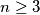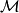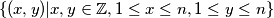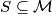### MEMO 2011 ekipno problem 3

Kvaliteta:
Avg: 3,0
Težina:
Avg: 6,0
For an integer$n \geq 3$, let$\mathcal M$ be the set$\{(x, y) | x, y \in \mathbb Z, 1 \leq x \leq n, 1 \leq y \leq n\}$ of points in the plane.

What is the maximum possible number of points in a subset$S \subseteq \mathcal M$ which does not contain three distinct points being the vertices of a right triangle?
Izvor: Srednjoeuropska matematička olimpijada 2011, ekipno natjecanje, problem 3